nextnano.com  GUI: nextnanomat  Tool: nextnano++  Tool: nextnano³  Tool: nextnano.NEGF  Download | Search | Copyright | News | Publications  * password protected nextnano³ software2D T-shaped strained QWR

# nextnano3 - Tutorial

## Electron and hole wave functions in a T-shaped strained quantum wire grown by CEO (cleaved edge overgrowth)

Author: Stefan Birner

If you want to obtain the input files that are used within this tutorial, please contact stefan.birner@nextnano.de.
``` -> 2DTshapedStrainedQuantumWireCEO.in  -> 2DTshapedStrainedQuantumWireCEO_no_piezo.in  -> 1DAlGaAs_InAlAs_QW.in```.

Acknowledgement: The author - Stefan Birner - would like to thank Robert Schuster from the University of Regensburg for providing the experimental data and some of the figures.

## T-shaped strained quantum wire

This tutorial treats strained quantum wires including a discussion of the strain calculation and the strain-induced piezoelectric fields (Poisson equation).

Similar to the 1D confinement in a quantum well, it is possible to confine electrons or holes in two dimensions, i.e. in a quantum wire.
The quantum wire is formed at the T-shaped intersection of a 10 nm GaAs type-I quantum well and a 10 nm In0.16Al0.84As barrier (!).
The T-shaped intersection is surrounded by Al0.3Ga0.7As which acts as a barrier to GaAs.
The In0.16Al0.84As barrier has a larger lattice constant than Al0.3Ga0.7As and is thus strained.
The strain affects the GaAs well and thus produces a local decrease (increase) in the conduction (valence) band edge energy and thus confines electrons (holes) at the T-shaped intersection.
The electrons and holes are free to move along the z direction only, thus the wire is oriented along the [0-11] direction.
Such a heterostructure can be manufactured by growing the layers along two different growth directions with the CEO (cleaved edge overgrowth) technique.

The following figure (upper part) shows the sample layout.
It is useful to compare it to the T-shaped quantum wire tutorial (lower part of the figure) which consists of two GaAs quantum wells rather than one GaAs well and one In0.16Al0.84As barrier in order to understand the fundamental difference between these two layouts.

 This tutorial (T-shaped strained quantum wire):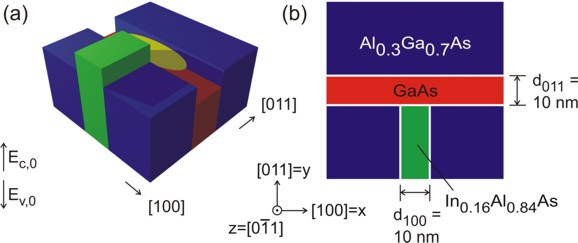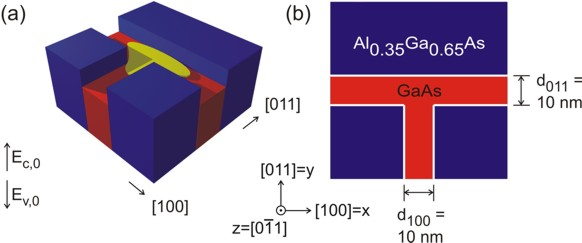(a) The figure at the top schematically shows the conduction band profile without considering strain effects (!) of the T-shaped wire that was grown by the CEO technique. If one inverts the energy arrow then the left picture corresponds to the valence band edge. The wave function is indicated at the T-shaped intersection in yellow. In the figure at the bottom, the wave function can extend into a larger volume (as compared to the quantum well) and thus reduce its energy. So quantum mechanics tells us that the ground state can be found at this intersection and electrons are only allowed to move one-dimensionally along the z direction. For the figure at the top, this is not true. The confinement only occurs if one takes into account the strain which decreases (increases) the conduction (valence) band edge energy in GaAs at the T-shaped intersection. (b) The figures on the right show a 60 nm x 60 nm extract of the schematic layout including the dimensions, the material composition and the orientation of the wire with respect to the crystal coordinate system.

It is sufficient to describe this heterostructure within a 2D simulation as it is translationally invariant along the z direction.
The simulation coordinate system is oriented in the following way:
```  \$domain-coordinates   domain-type        = 1  1  0  ! ```(x,y) plane```   hkl-x-direction-zb = 1  0  0  ! ```along the`   `direction in the crystal coordinate system```   hkl-y-direction-zb = 0  1  1  ! ```along the`   `direction in the crystal coordinate system``` ! hkl-z-direction-zb = 0 -1  1  ! ``` along the` [0-11] `direction in the crystal coordinate system``` ```The` hkl-z-direction `does not have to be specified. It is calculated internally in the code.

The simulations are performed at 4 Kelvin:
``` \$global-parameters   lattice-temperature = 4.0d0   ! [K]```

The lattice constants are temperature dependent.
` \$numeric-control`
```  lattice-constants-temp-coeff-on = yes ! temperature dependent lattice constants```

Our sample is simulated on a domain which has an extension of 310 nm along the x direction and 410 nm along the y direction.

### Calculation of the strain tensor

First, we have to calculate the strain tensor by minimizing the elastic energy within continuum elasticity theory.
Along the translationally invariant z direction the lattice commensurability constraint forced the In0.16Al0.84As layer to adopt the lattice constant of Al0.3Ga0.7As.

``` \$simulation-flow-control   ...   strain-calculation = strain-minimization```

``` \$strain-minimization-model           ! ```for``` "strain-calculation = strain-minimization"   substrate-cluster-number = 4        ! ```Here one specifies the relevant cluster that acts as the (unstrained) substrate. (here: Al0.3Ga0.7As)```   boundary-condition-x     = Neumann  ! ```This corresponds to 'no external forces acting on the sample', i.e. the derivative of the stress tensor is zero.```   boundary-condition-y     = Neumann  ! ```This corresponds to 'no external forces acting on the sample', i.e. the derivative of the stress tensor is zero.```   boundary-condition-z     = periodic ! ```redundant, only necessary for 3D simulation```  ```

The hydrostatic strain (trace of the strain tensor, i.e. ehydro = exx + eyy + ezz), i.e. relative change in volume) has its maximum at the intersection,
where it leads to a reduced band gap which is the requirement for confining the charge carriers.
Thus, the quantum wire is formed in the GaAs quantum well due to the tensile strain field induced by the In0.16Al0.84As layer.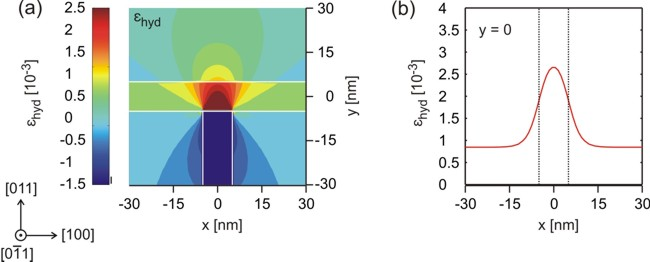(a) The figure shows the hydrostatic strain. It can be cleary seen that the maximum volume deformation (red: tensile strain) occurs at the T-shaped intersection, thus reducing the band gap at this position and providing a confinement for both electrons and holes. (b) This is a cut through figure (a) at position y=0. The data shwon in this plot can be found in the file:``` strain/e_hydro2D_slice_middle_range_along_x.dat``` Note: The scale on the left figure is wrong. The dark blue area should have the value -15 rather than -1.5. The reason is due to the color map. All values below -1.5 have the same color (dark blue).

Note: In a one-dimensional example, the strain tensor components of an In0.16Al0.84As layer that is strained pseudomorphically with respect to an Al0.3Ga0.7As substrate are the following:

`exx = 0.01096 = 11 * 10-3`

`eyy = ezz = -0.01245 = -12 * 10-3`

`exy = exz = eyz = 0`

`ehydro = Tr(eij) = -0.01393 = -14 * 10-3`

The growth direction is along the x direction, i.e. along . The relevant input file is` 1DAlGaAs_InAlAs_QW.in`.
These strain tensor components can be found in the file:``` strain/strain_cr1D.dat```
If you want to obtain this input file, please contact stefan.birner@nextnano.de.
The temperature is assumed to be 4 Kelvin and the lattice constants are assumed to be temperature dependent (i.e. we use the 4 K lattice constants).

The individual strain tensor components (`exx`, `eyy`, `exy`) with respect to the simulation coordinate system look like this: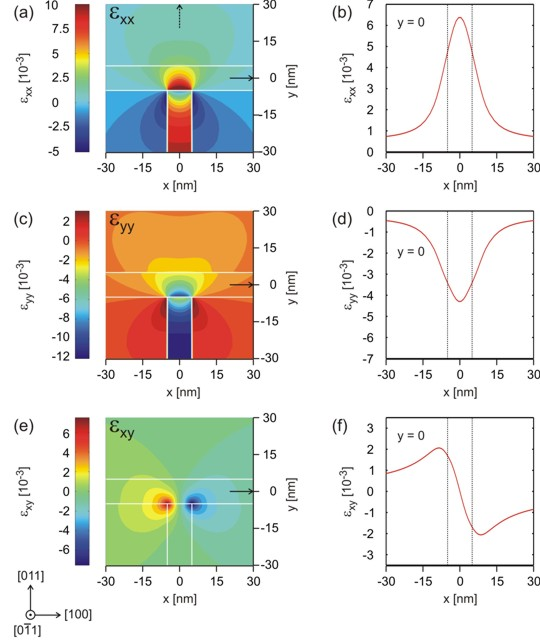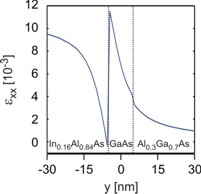(a), (c), (e) The color maps on the left show the strain tensor components exx, eyy and exy. (b), (d), (f) show a cut through the color maps for y=0. The picture at the upper right corner shows the cut for x=0 of the strain tensor component exx.

In our 2D simulation, our sample layout is homogeneous along the z direction, i.e. the lattice constant of In0.16Al0.84As is forced to have the same lattice constant as Al0.3Ga0.7As along the z direction. Then the strain tensor component must be``` ezz = -0.01245```, in agreement with our 1D example, i.e. In0.16Al0.84As which has a larger lattice constant than Al0.3Ga0.7As is strained compressively along the z direction. Similar to the 1D case, it is also expected that the` eyy `component inside the In0.16Al0.84As barrier has a similar value to ` ezz`, which is clearly the case. The dark blue area in Fig. (c) thus has a value around` -12 * 10-3`. However, this value deviates from the ideal 1D value at the T-shaped intersection as expected. The same applies to the value of` exx `which is similar to the 1D value inside the In0.16Al0.84As barrier:` exx = 11 * 10-3`

The strain tensor components `exz `and``` eyz ```with respect to the simulation coordinate system re equal to zero as in our 1D example.

The important difference with respect to the 1D case is the existence of a non-vanishing strain tensor component` exy `which brakes the symmetry of the sample layout.
Usually, the` exy `component is attributed to be responsible for piezoelectricity. However, note that in these comments, all strain tensor components refer to the simulation coordinate system (and not to the crystal coordinate system). So we have to plot the off-diagonal strain tensor components that are expressed with respect the crystal coordinate system orientation and then check if the off-diagonal components are non-zero which clearly is the case.The color maps show the strain tensor components exxcr, eyycr, exycr=exzcr and eyzcr with respect to the crystal coordinate system. The rotation with respect to the simulation system is a rotation of 45 degrees around the x axis, i.e. the  axis.

Clearly, Fig. (a) shows that exxcr = exx because the x coordinate axes coincide. Symmetry arguments show that the following holds:

eyycr = 1/2 ( eyy + ezz )

exycr = exzcr = 1/SQRT(2) exy

### Calculation of the piezoelectric charge density

The off-diagonal strain tensor components exycr, exzcr and eyzcr are responsible for the piezoelectric polarization where e14 is the piezoelectric constant in units of [C/m²]:

( 2 eyzcr )
Ppiezo = e14   ( 2 exzcr )
( 2 exycr )

Once having determined the piezoelectric polarization Ppiezo one is able to compute the piezoelectric charge density: ρpiezo(x) = `-` div Ppiezo(x).

The strain-induced piezoelectric fields are then obtained by solving Poisson's equation.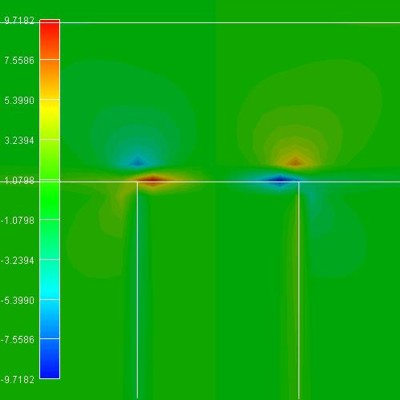The piezoelectric charge density in units of 1 * 1018 e/cm³.

### Calculation of the conduction and valence band edges

The new conduction and valence band edges were determined by taking into account the shifts and splittings due to the relevant deformation potentials as well as the changes due to the piezoelectric fields.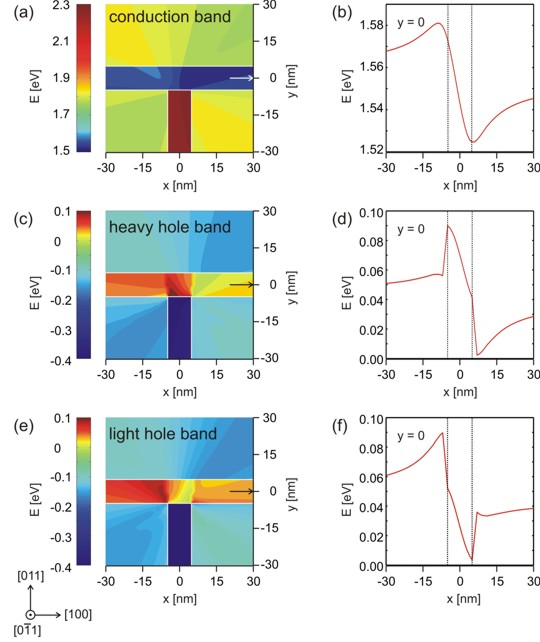(a), (c), (e) Color maps of the conduction, heavy hole and light hole band edge energies. (b), (d), (f) A cut through the conduction, heavy hole and light hole band edge energies at y=0. Very interestingly, one can see that the electron feels a conduction band minimum which is located left with respect to the T-shaped intersection. The valence band maximum for the heavy hole is not at the same position as the valence band maximum for the light hole.

### Electron and heavy hole wave functions

The size of the quantum cluster is 110 nm x 110 nm.

The following figure shows the square of the electron (e) and heavy hole (hh) wave functions (i.e. psi²). They were calculated within the effective-mass approximation (single-band). For the holes we derived the effective mass tensor from the Luttinger parameters to include anisotropy. For the upper part of the figure the piezo effect was not included. As one can clearly see in the lower part of the figure, the piezo effect destroys the symmetry of the sample layout. The piezoelectric field results from the exy strain tensor component which is also not symmetric with respect to the T-shaped geometry.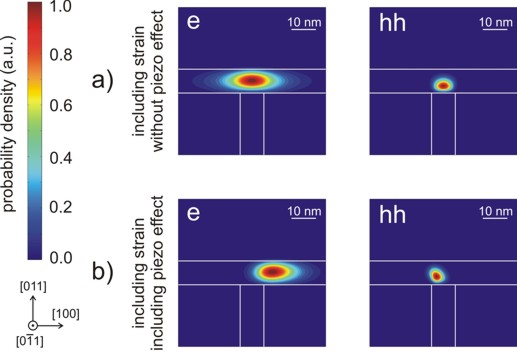a) Contour diagram of the square of the electron (e) and heavy hole (hh) wave functions (i.e. psi²) for the case where strain is included in the simulations but piezoelectricity is not. b) Same as in a) but this time including the piezo effect. Note: Here, the wave functions are normalized so that the maximum of each equals one. The size of the shown squares is 60 nm x 60 nm whereas the size of the quantum cluster was chosen to be 110 nm x 110 nm.

More details can be found in the following PhD thesis:

Hochortsaufgelöste optische Spektroskopie an niedrigdimensionalen Halbleiterstrukturen
Robert Schuster
University of Regensburg (2005)

In this thesis the "confinement energy" is defined as the difference between the transition energies of the quantum wire and the quantum well.
They can be calculated with the nextnano software as a function of alloy content or well widths.
In this thesis, excitonic effects were not included.

• Please help us to improve our tutorial. Send comments to``` support [at] nextnano.com```.